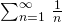# An interesting time complexity question

What is the time complexity of following function fun()?

 `int` `fun(``int` `n) ` `{     ` `    ``for` `(``int` `i = 1; i <= n; i++) ` `    ``{ ` `        ``for` `(``int` `j = 1; j < n; j += i) ` `        ``{ ` `            ``// Some O(1) task ` `        ``} ` `    ``}     ` `} `

For i = 1, the inner loop is executed n times.
For i = 2, the inner loop is executed approximately n/2 times.
For i = 3, the inner loop is executed approximately n/3 times.
For i = 4, the inner loop is executed approximately n/4 times.
…………………………………………………….
…………………………………………………….
For i = n, the inner loop is executed approximately n/n times.

So the total time complexity of the above algorithm is (n + n/2 + n/3 + … + n/n)

Which becomes n * (1/1 + 1/2 + 1/3 + … + 1/n)

The important thing about series (1/1 + 1/2 + 1/3 + … + 1/n) is, it is equal to Θ(Logn) (See this for reference). So the time complexity of the above code is Θ(nLogn).

As a side note, the sum of infinite harmonic series is counterintuitive as the series diverges. The value ofis ∞. This is unlike geometric series as geometric series with ratio less than 1 converges.